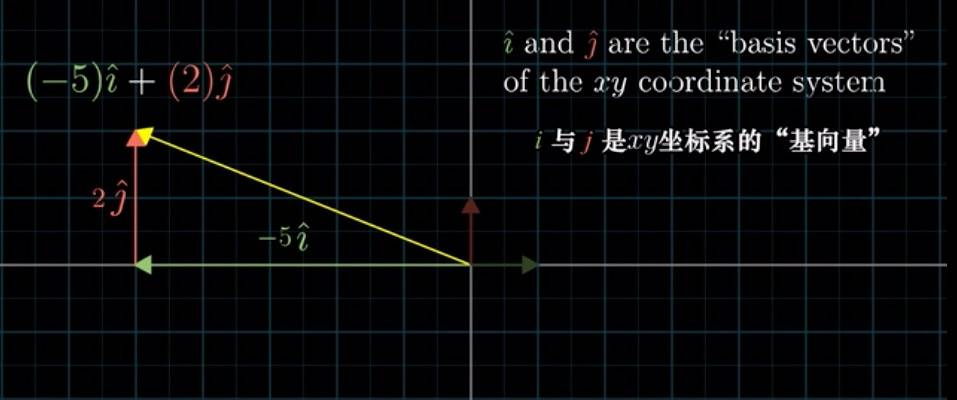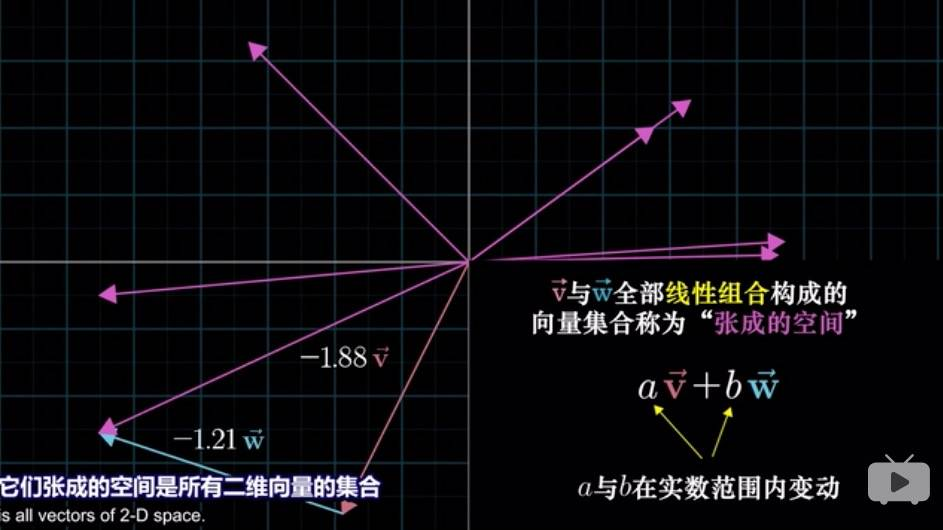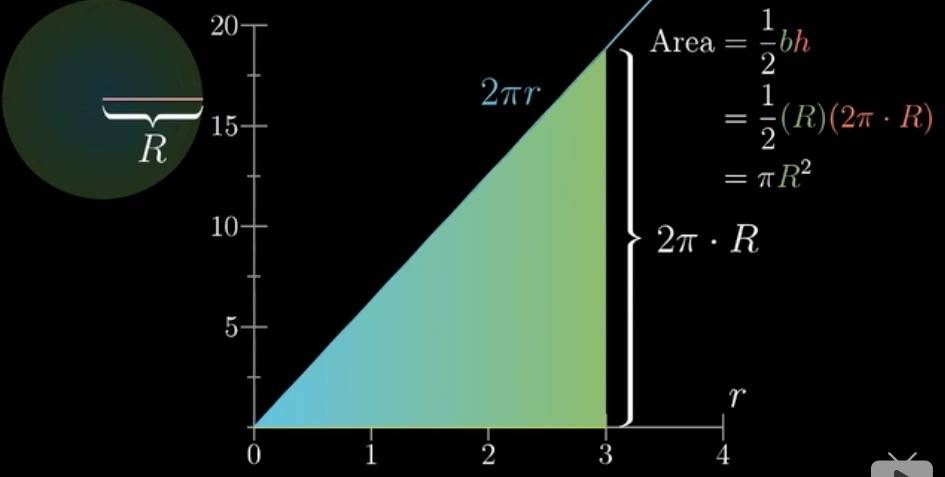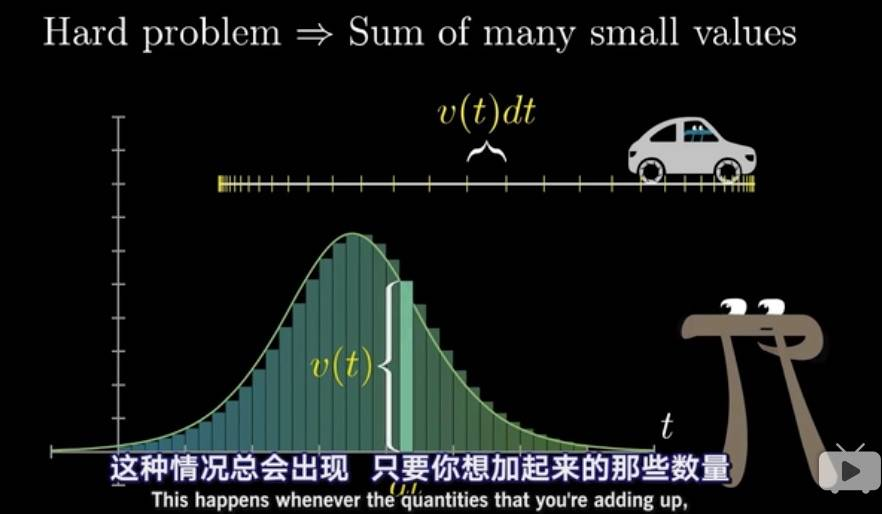# 目录

1. 程序设计与 C 语言
1.1 计算机和编程语言
1.2 C 语言
1.3 第一个程序
2. 计算
2.1 变量
2.2 数据类型
2.3 表达式
3. 判断与循环
3.1 判断
3.2 循环
4. 进一步的判断与循环
4.1 逻辑类型和运算
4.2 级联和嵌套的判断
4.3 多路分支
4.4 循环的例子
4.5 判断和循环常见的错误
5. 循环控制
5.1 循环控制
5.2 多重循环
5.3 循环应用
6. 数组与函数
6.1 数组
6.2 函数的定义与使用
6.3 函数的参数和变量
6.4 二维数组
7. 数组运算
7.1 数组运算
7.2 搜索
7.3 排序初步
8. 指针与字符串
8.1 指针
8.2 字符类型
8.3 字符串
8.4 字符串计算

# 1. 程序设计与 C 语言

## 1.1 计算机和编程语言

• 人：What to do；计算：How to do
• 计算机做的所有事情都是计算，计算的步骤就是算法。
• 程序的执行
• 解释
• 编译

## 1.2 C 语言

• 历史

• FORTRAN - BCPL - B - C
• 1973 年 3 月：第三版 Unix 上出现了 C 语言编译器
• 1973 年 11 月：第四版 Unix 完全用 C 语言重写
• 版本

• 经典 C

• 1989 ANSI C

• 1990 ISO 接受了 ANSI 的标准——C89

• 1995 1999 两次更新：C95 C99

• 应用
• 操作系统
• 嵌入式系统
• 驱动程序
• 底层驱动：图形引擎、图像处理、声音效果等

# 线性组合、张成的空间与基Trick：单个向量看作箭头；多个向量看作点（否则会太拥挤）。所以对大部分二维向量对来说，它们张成的空间是整个无限大的二维平面；但如果共线，它们张成的空间就是一条直线。

# 导论（难怪正态分布这么多见……）f(x) 可以是任意函数

# 机器学习概念

1. 由于作了一些简化，可能会与上面链接中的内容不太一致。
2. GitHub 中公式无法渲染，下载到本地即可，推荐用 Typora — a markdown editor, markdown reader.

# 背景

• 来源：复旦大学公开课：人工智能哲学_全 7 集_网易公开课
• 讲师：徐英瑾教授
• 课程介绍：本课程从人工智能科学发展的科学史概要出发，讨论了哲学思辨和人工智能研究之间的密切关系，并从人工智能的角度，重新审视了近代欧洲哲学对于 “机器是否能够思维” 这个问题的思辨结论。尔后，讨论了如何从当代计算机科学的角度来解读康德哲学，并从中得到一个关于类比推理的计算模型。本课程也讨论了当代美国哲学家塞尔对于 “计算模型如何获得关于符号的语义知识” 的忧虑，并进一步探讨了这一忧虑在计算机科学内部的表达形式：框架问题。

• 文章目录
1 简介
2 证明
2.1 概念与过程形式化
2.2 理论推导
2.3 证明
2.3.1 最优判别器
2.3.2 最优生成器
2.4 重述训练过程
2.4.1 参数优化过程
2.4.2 实际训练过程
2.4.3 最终过程
3 代码
4 代码学习
5 应用
6 数据集
7 其他资料
8 参考文献

# Language Model Note (SLP Ch03)

## Ngram

• Count up the frequency and divide (use maximum likelihood estimation or MLE):

• Example: $P(you | i\ love) := \frac {c(i\ love\ you)}{c(i\ love)}$
• More Generally: $P(w_1^n) = P(w_1)P(w_2 | w_1)P(w_3|w_1^2)...P(w_n|w_1^{n-1}) = \prod_{i=1}^{n} P(w_i|w_1^{i-1})$
• For N-gram

• Next Word: $P(w_n|w_1^{n-1}) \approx P(w_n|w_{n-N+1}^{n-1}) = \frac {C(w_{n-N+1}^{n-1}w_n)}{C(w_{n-N+1}^{n-1})}$
• prob of sentence: $P(w_1^n) \approx \prod_{i=1}^{n} P(w_i|w_{i-1}...w_{i-N+1})$
• For Bigram
• prob of sentence: $P(w_1^n) \approx \prod_{i=1}^{n} P(w_i|w_{i-1})$
• Next Word: $P(w_n|w_{n-1}) = \frac {C(w_{n-1}w_n)}{\sum_w C(w_{n-1} w)} = \frac {C(w_{n-1}w_n)}{C(w_{n-1})}$